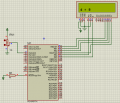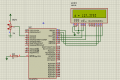# wanted to map adc into 0-255 range.But code not working

Thread Starter

#### khatus

Joined Jul 2, 2018
89
Hello guys i wanted to map adc value (0-1023) to 0-255 range. But the code did not working on proteus 8.6.I have created a map function for this.Here is my code.But can not guess where is my fault?

Code:
sbit LCD_RS at RB4_bit;
sbit LCD_EN at RB5_bit;
sbit LCD_D4 at RB0_bit;
sbit LCD_D5 at RB1_bit;
sbit LCD_D6 at RB2_bit;
sbit LCD_D7 at RB3_bit;

sbit LCD_RS_Direction at TRISB4_bit;
sbit LCD_EN_Direction at TRISB5_bit;
sbit LCD_D4_Direction at TRISB0_bit;
sbit LCD_D5_Direction at TRISB1_bit;
sbit LCD_D6_Direction at TRISB2_bit;
sbit LCD_D7_Direction at TRISB3_bit;

float map(unsigned int value, unsigned int min, unsigned int max)//function definition
{
return(((max - min)/1023)*value);
}

char Txt;
void main()
{
unsigned int adc_value;
float d;
PORTA = 0b00000000;
TRISA = 0xFF;
ADC_Init();
Lcd_Init();                        // Initialize LCD
Lcd_Cmd(_LCD_CLEAR);               // Clear display
Lcd_Cmd(_LCD_CURSOR_OFF);          // Cursor off

while(1)
{
adc_value = ADC_read(0);
d = map(adc_value,0,255);
FloatToStr(d,Txt);    // Convert voltage to string
Lcd_Out(2,1,"d =");
Lcd_Out(2,5,Txt);
Delay_ms(300);
}

}Last edited:

#### AlbertHall

Joined Jun 4, 2014
11,313
Thread Starter

#### khatus

Joined Jul 2, 2018
89
Thanks it worksCode:
sbit LCD_RS at RB4_bit;
sbit LCD_EN at RB5_bit;
sbit LCD_D4 at RB0_bit;
sbit LCD_D5 at RB1_bit;
sbit LCD_D6 at RB2_bit;
sbit LCD_D7 at RB3_bit;

sbit LCD_RS_Direction at TRISB4_bit;
sbit LCD_EN_Direction at TRISB5_bit;
sbit LCD_D4_Direction at TRISB0_bit;
sbit LCD_D5_Direction at TRISB1_bit;
sbit LCD_D6_Direction at TRISB2_bit;
sbit LCD_D7_Direction at TRISB3_bit;

float map(int val, int in_min, int in_max, int out_min, int out_max) {
return (float)(val - in_min) * (out_max - out_min) / (in_max - in_min) + out_min;
}

char Txt;
void main()
{
unsigned int adc_value;
float d;
PORTA = 0b00000000;
TRISA = 0xFF;
ADC_Init();
Lcd_Init();                        // Initialize LCD
Lcd_Cmd(_LCD_CLEAR);               // Clear display
Lcd_Cmd(_LCD_CURSOR_OFF);          // Cursor off

while(1)
{
adc_value = ADC_read(0);
d = map(adc_value,0,1023,0,255);
FloatToStr(d,Txt);    // Convert voltage to string
Lcd_Out(2,1,"d =");
Lcd_Out(2,5,Txt);
Delay_ms(300);
}  }#### MrChips

Joined Oct 2, 2009
23,535
Seems like a complicated way to remove two bits.
Why not this?

new_value = adc_value >> 2;

#### AlbertHall

Joined Jun 4, 2014
11,313
TS opted to use a float value.

#### MrChips

Joined Oct 2, 2009
23,535

#### BobTPH

Joined Jun 5, 2013
3,316
There is s setting in the PIC ADCs that puts the 8 high bits of the result in a single byte, so you can do it wirh no code at all!

Bob

Last edited:
Similar threads